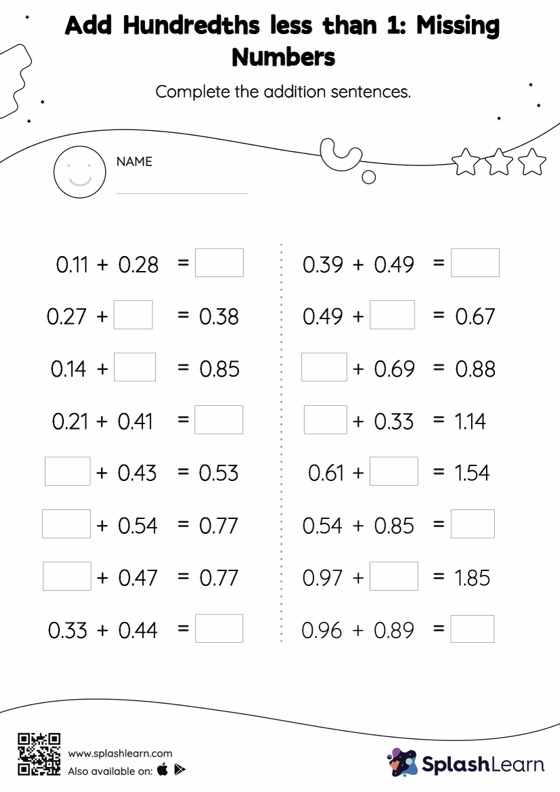# Add Hundredths less than 1: Missing Numbers Worksheet

Home > Add Hundredths less than 1: Missing NumbersThis add hundredths less than 1 worksheet consists of tasks to help your young mathematician develop fluency with addition. When adding decimals on this worksheet, students align the decimal points and use zero as a placeholder. Then to find the missing number in the add hundredths less than 1 worksheet, students then apply the relationship between addition and subtraction.